# RD Sharma Solutions For Class 7 Maths Chapter 21 Mensuration - II (Area of Circle) Exercise 21.2

The PDF of RD Sharma Solutions for Class 7 Exercise 21.2 of Chapter 21 Mensuration – II (Area of Circle) is given below. Learners can access and download the PDF for free. The solutions to this exercise come with detailed explanations structured by our expert teachers that further makes learning and understanding of concepts an easy task. RD Sharma Solutions for Class 7 textbook includes the concepts in a detailed and step by step way to help the students understand effectively. This exercise includes the area of the circle.

## Download the PDF of RD Sharma Solutions For Class 7 Maths Chapter 21 – Mensuration – II (Area of Circle) Exercise 21.2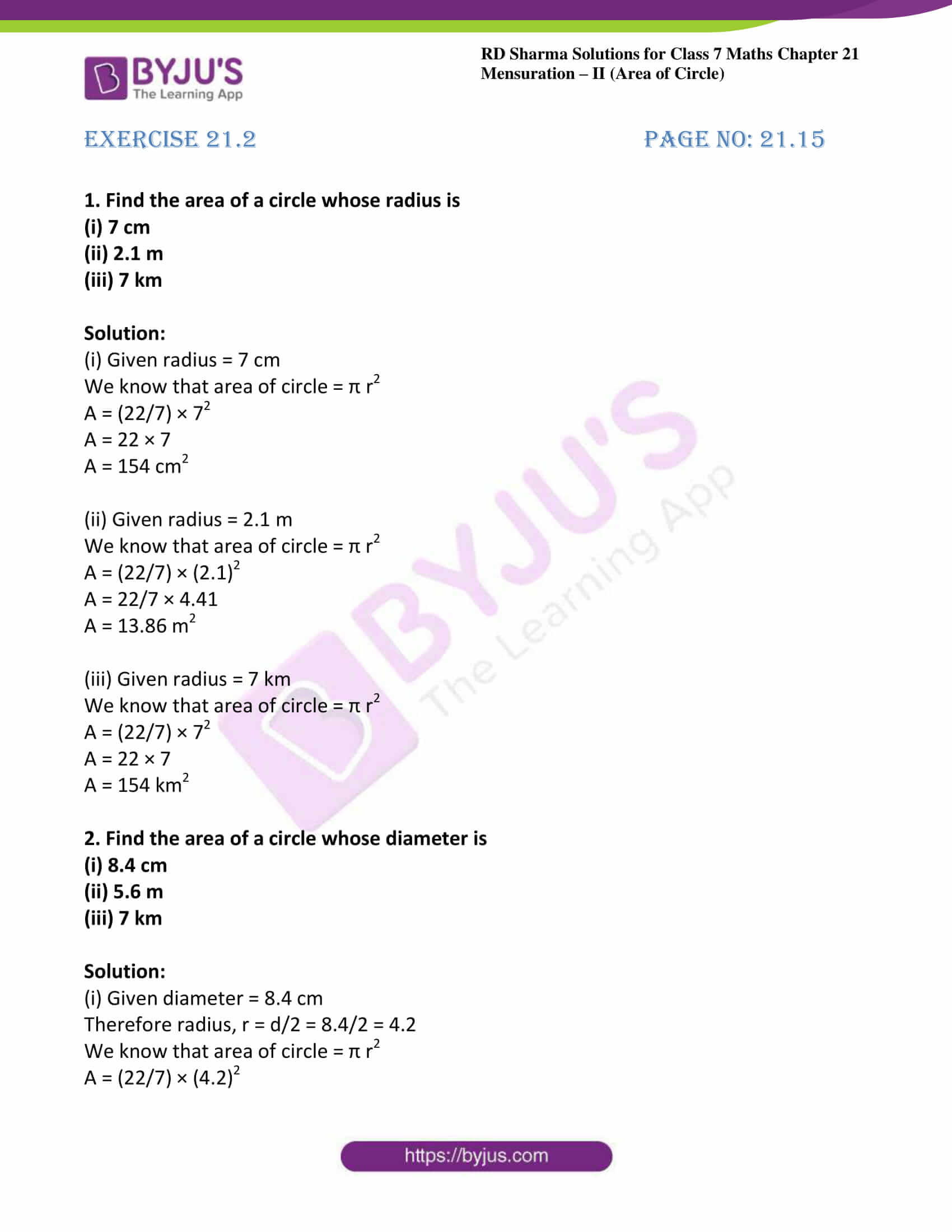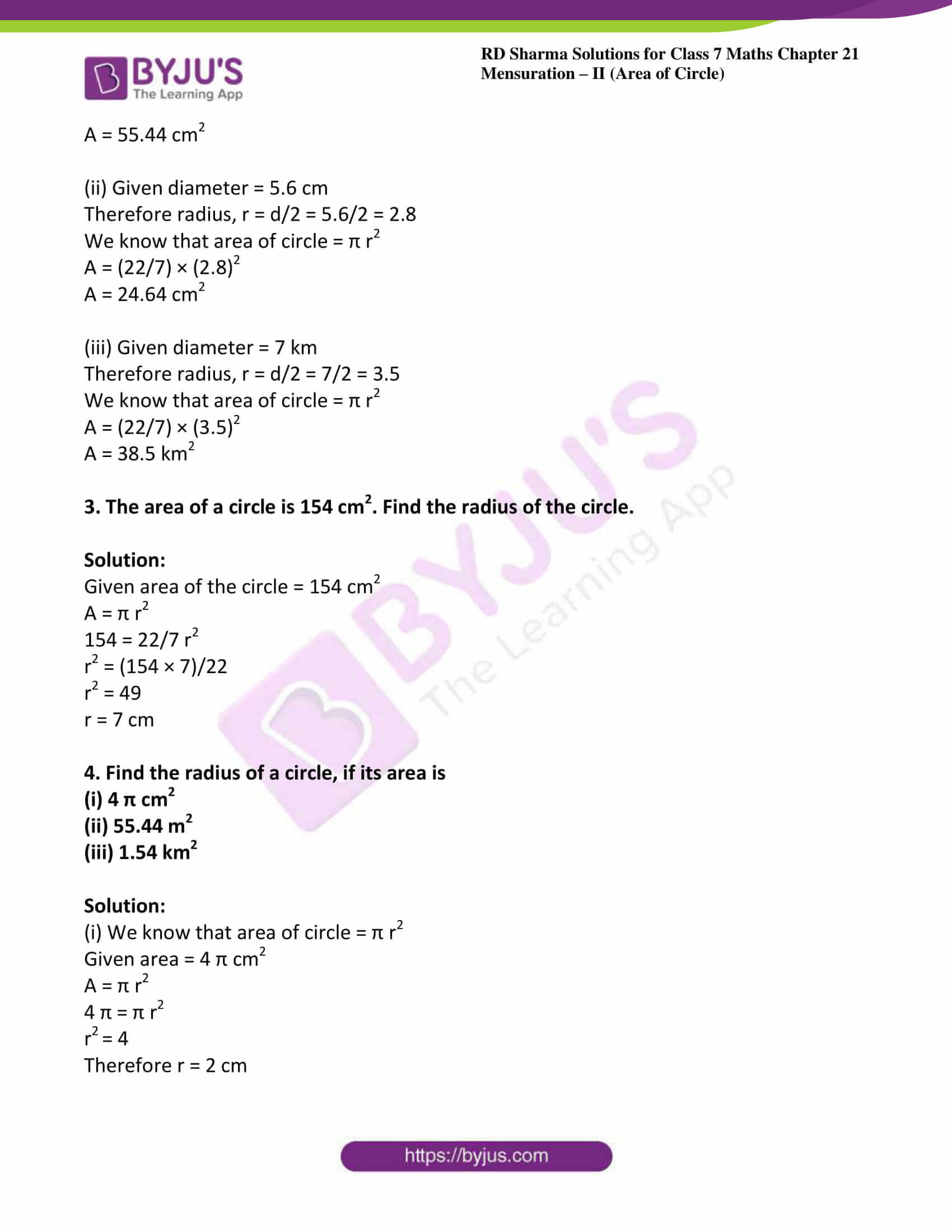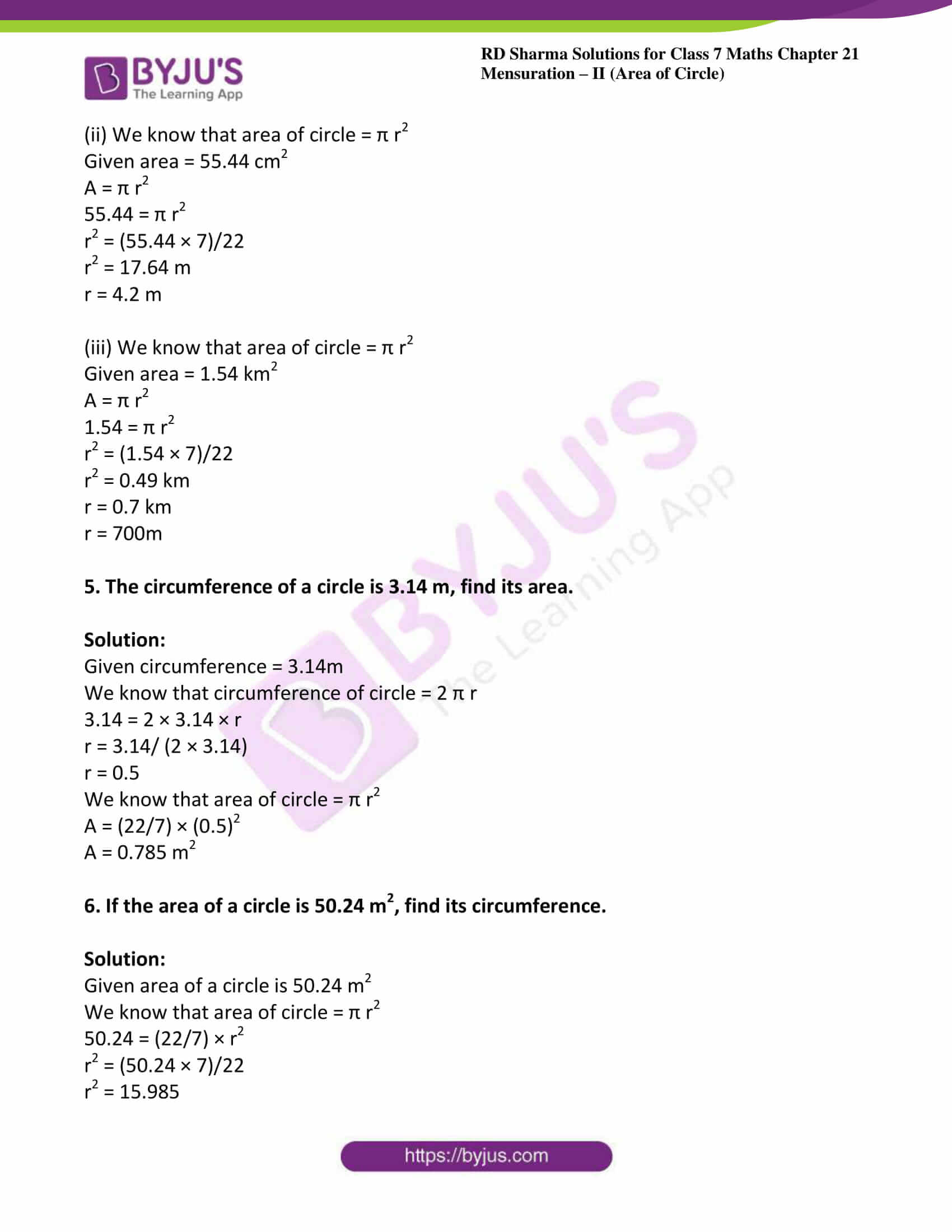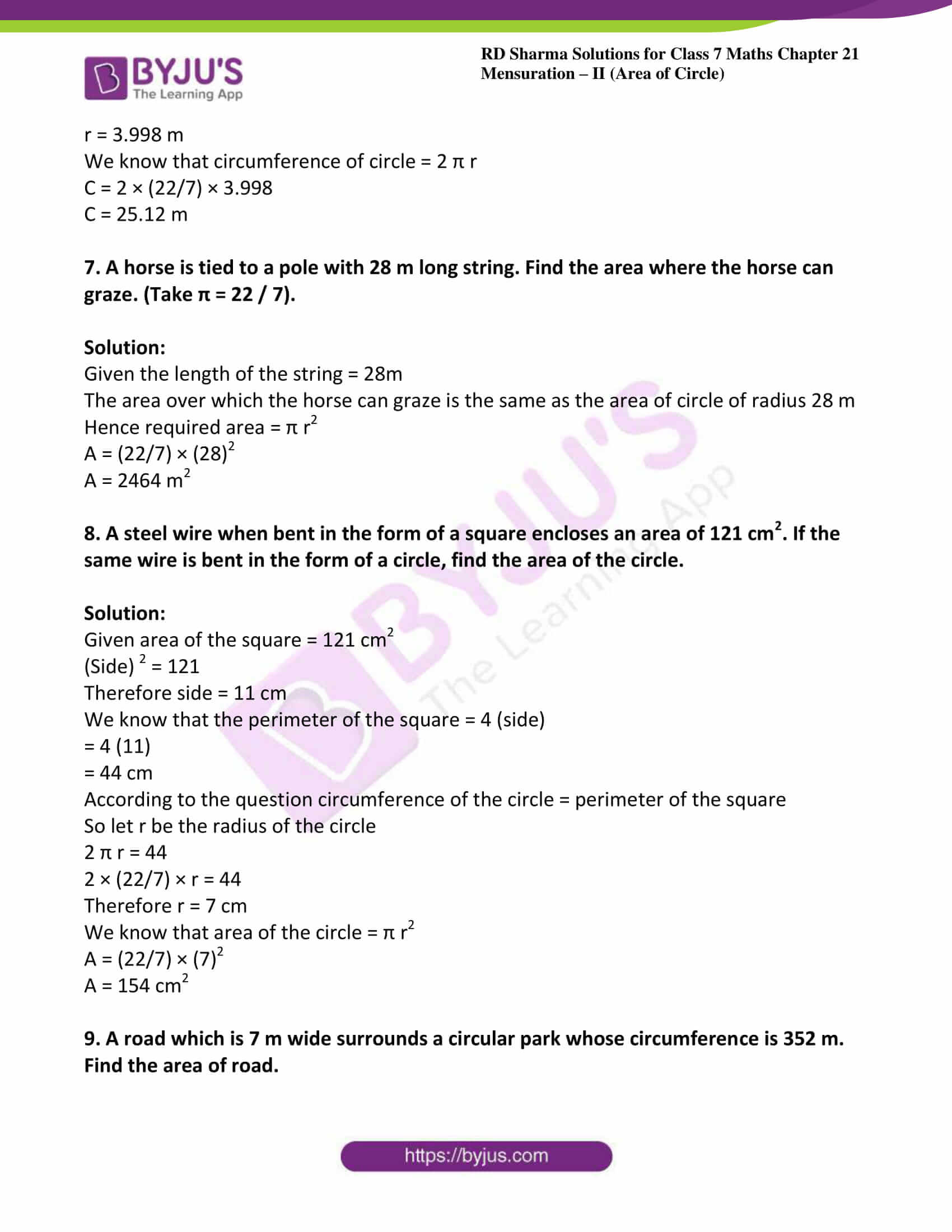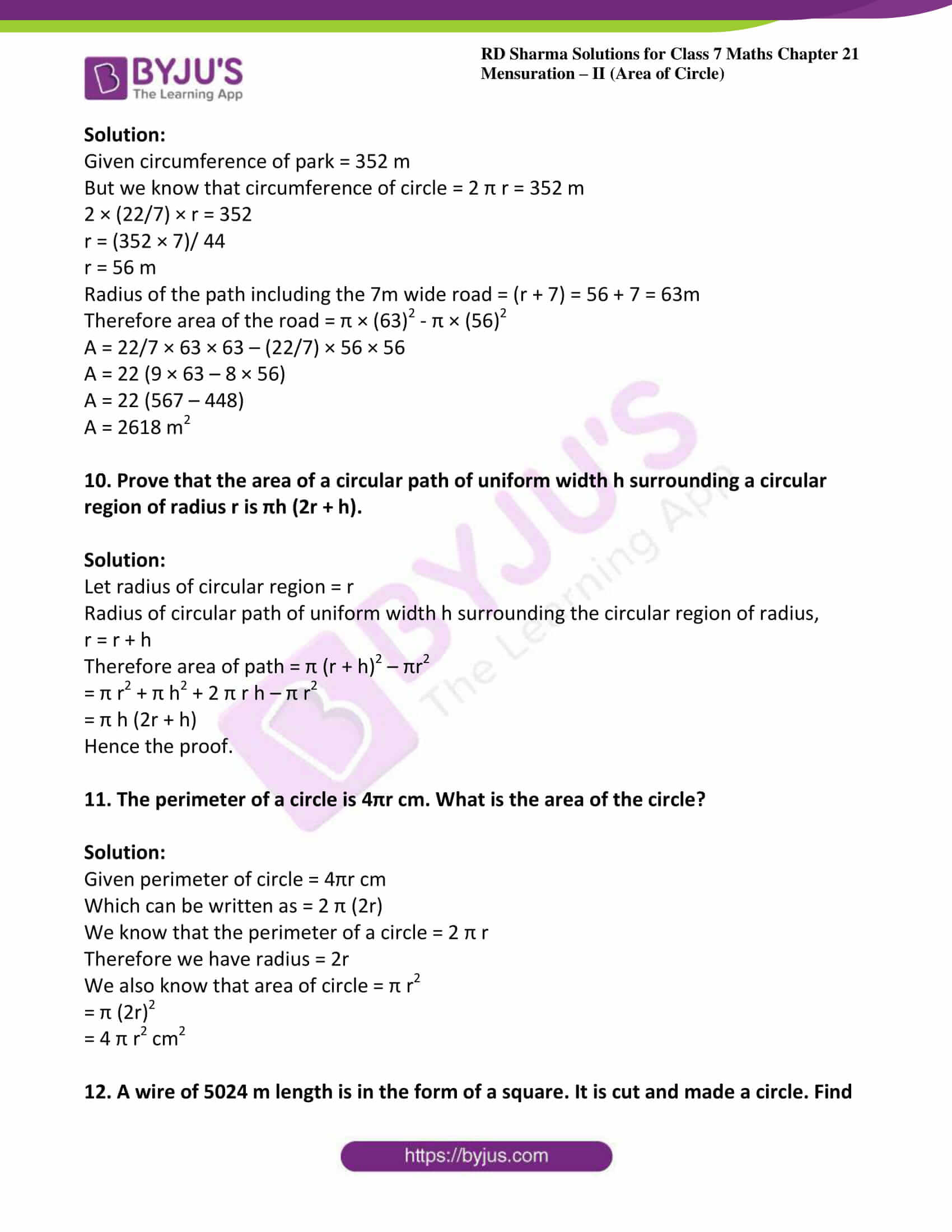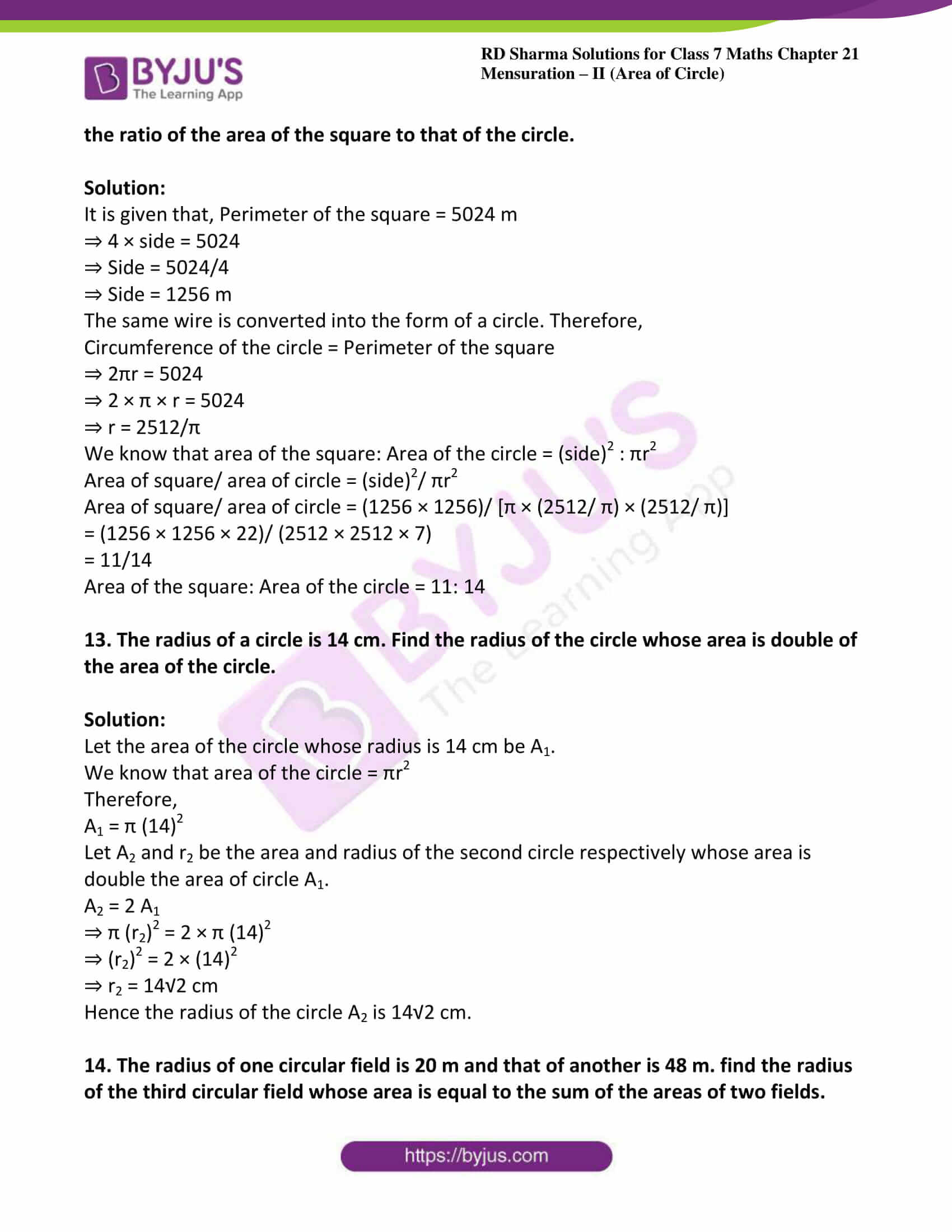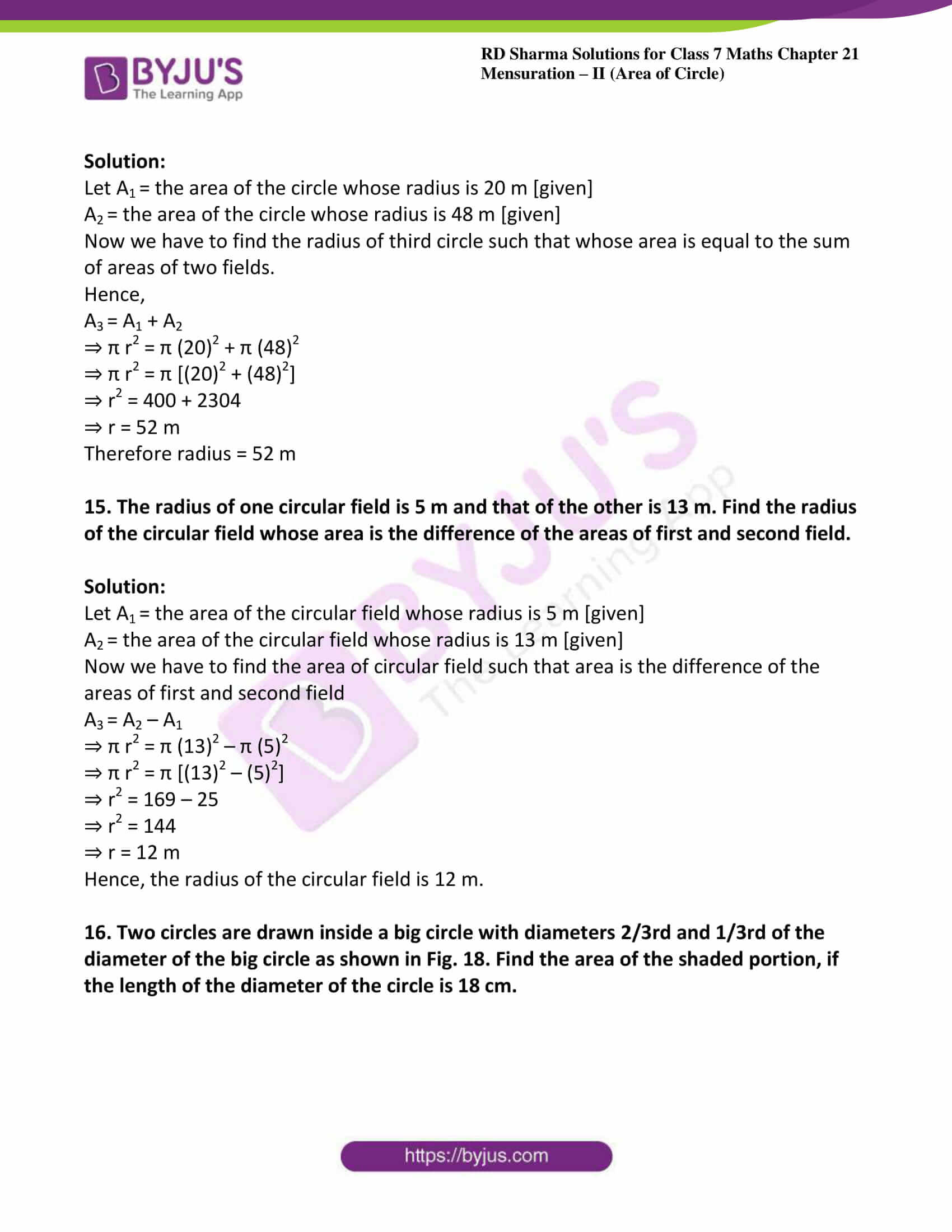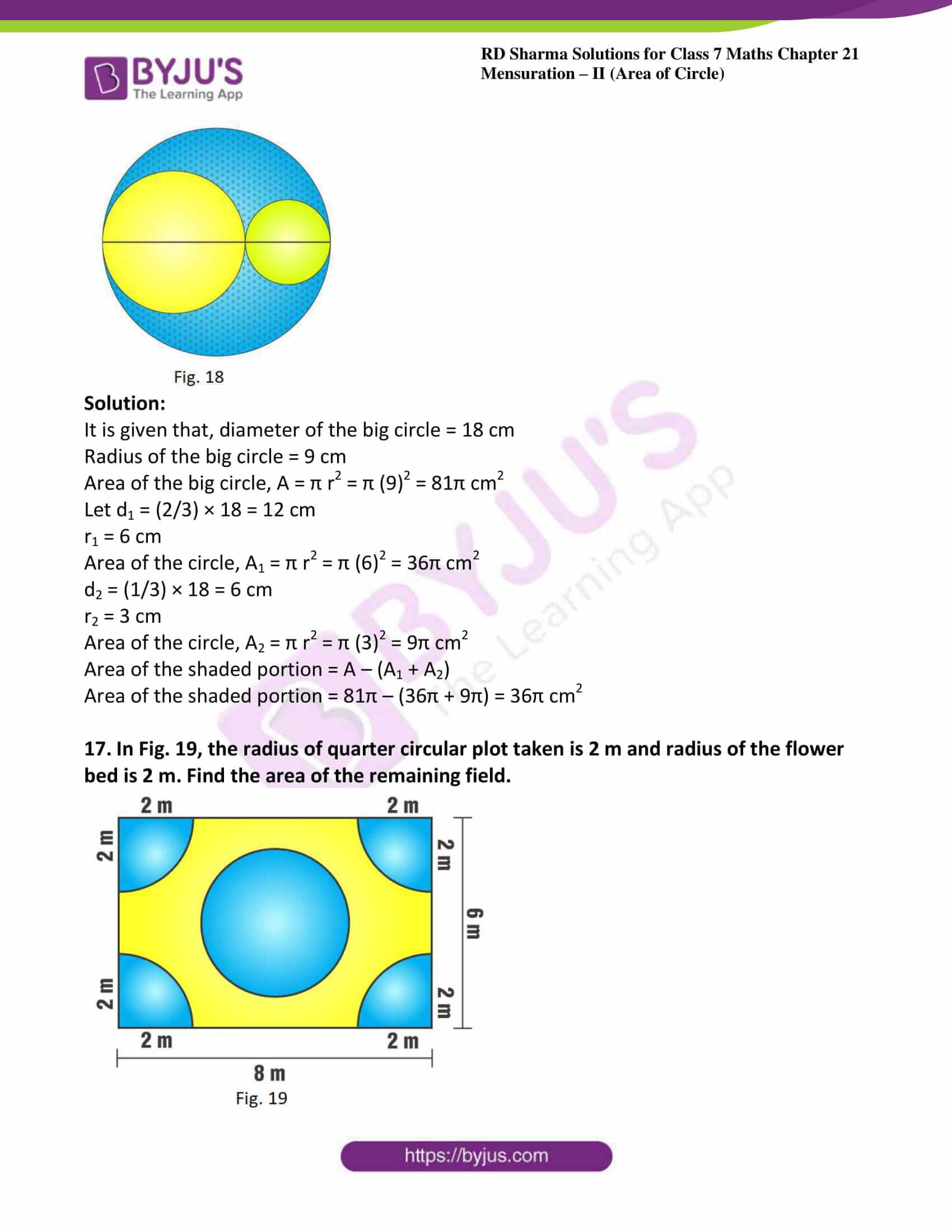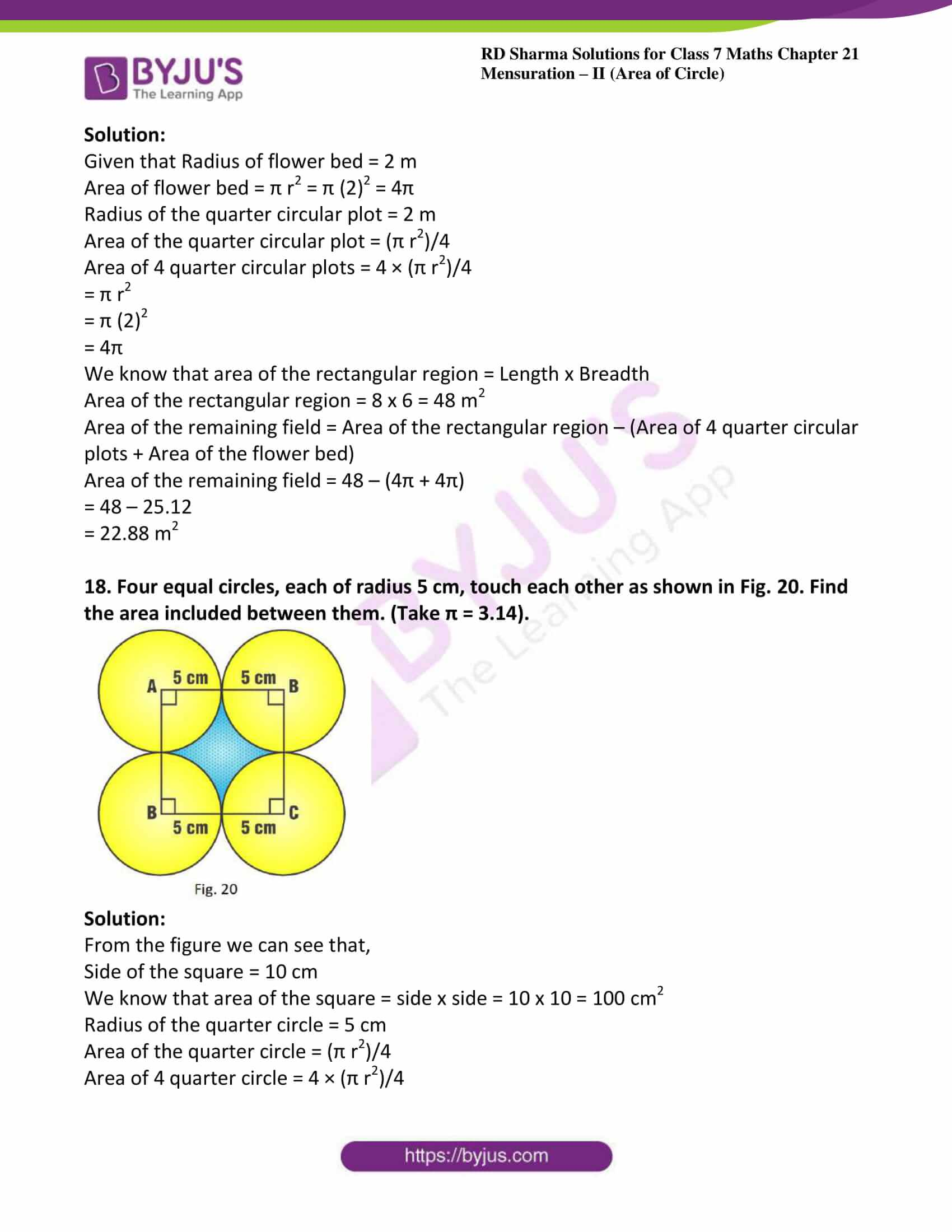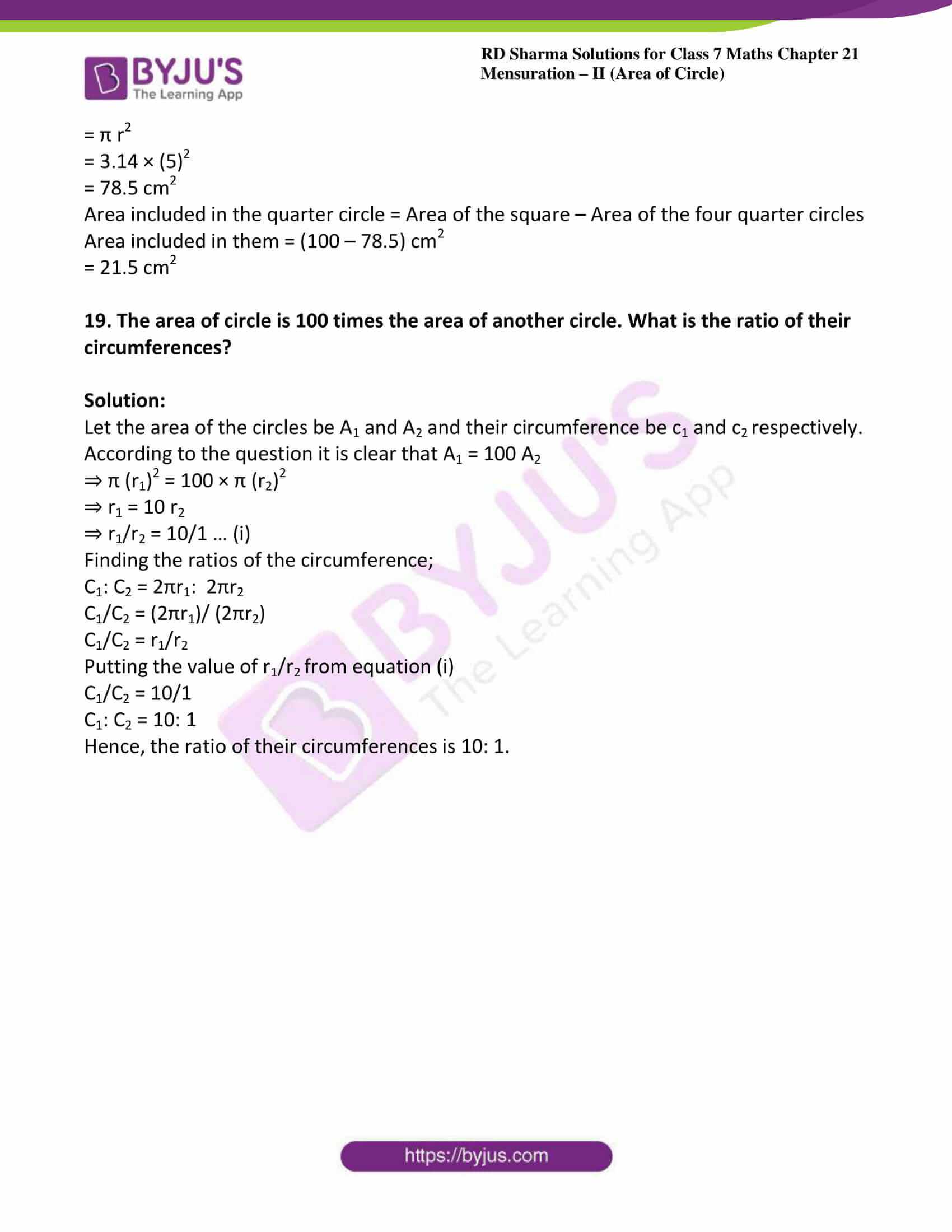### Access answers to Maths RD Sharma Solutions For Class 7 Chapter 21 – Mensuration – II (Area of Circle) Exercise 21.2

Exercise 21.2 Page No: 21.15

1. Find the area of a circle whose radius is

(i) 7 cm

(ii) 2.1 m

(iii) 7 km

Solution:

(i) Given radius = 7 cm

We know that area of circle = π r2

A = (22/7) × 72

A = 22 × 7

A = 154 cm2

(ii) Given radius = 2.1 m

We know that area of circle = π r2

A = (22/7) × (2.1)2

A = 22/7 × 4.41

A = 13.86 m2

(iii) Given radius = 7 km

We know that area of circle = π r2

A = (22/7) × 72

A = 22 × 7

A = 154 km2

2. Find the area of a circle whose diameter is

(i) 8.4 cm

(ii) 5.6 m

(iii) 7 km

Solution:

(i) Given diameter = 8.4 cm

Therefore radius, r = d/2 = 8.4/2 = 4.2

We know that area of circle = π r2

A = (22/7) × (4.2)2

A = 55.44 cm2

(ii) Given diameter = 5.6 cm

Therefore radius, r = d/2 = 5.6/2 = 2.8

We know that area of circle = π r2

A = (22/7) × (2.8)2

A = 24.64 cm2

(iii) Given diameter = 7 km

Therefore radius, r = d/2 = 7/2 = 3.5

We know that area of circle = π r2

A = (22/7) × (3.5)2

A = 38.5 km2

3. The area of a circle is 154 cm2. Find the radius of the circle.

Solution:

Given area of the circle = 154 cm2

A = π r2

154 = 22/7 r2

r2 = (154 × 7)/22

r2 = 49

r = 7 cm

4. Find the radius of a circle, if its area is

(i) 4 π cm2

(ii) 55.44 m2

(iii) 1.54 km2

Solution:

(i) We know that area of circle = π r2

Given area = 4 π cm2

A = π r2

4 π = π r2

r2 = 4

Therefore r = 2 cm

(ii) We know that area of circle = π r2

Given area = 55.44 cm2

A = π r2

55.44 = π r2

r2 = (55.44 × 7)/22

r2 = 17.64 m

r = 4.2 m

(iii) We know that area of circle = π r2

Given area = 1.54 km2

A = π r2

1.54 = π r2

r2 = (1.54 × 7)/22

r2 = 0.49 km

r = 0.7 km

r = 700m

5. The circumference of a circle is 3.14 m, find its area.

Solution:

Given circumference = 3.14m

We know that circumference of circle = 2 π r

3.14 = 2 × 3.14 × r

r = 3.14/ (2 × 3.14)

r = 0.5

We know that area of circle = π r2

A = (22/7) × (0.5)2

A = 0.785 m2

6. If the area of a circle is 50.24 m2, find its circumference.

Solution:

Given area of a circle is 50.24 m2

We know that area of circle = π r2

50.24 = (22/7) × r2

r2 = (50.24 × 7)/22

r2 = 15.985

r = 3.998 m

We know that circumference of circle = 2 π r

C = 2 × (22/7) × 3.998

C = 25.12 m

7. A horse is tied to a pole with 28 m long string. Find the area where the horse can graze. (Take π = 22 / 7).

Solution:

Given the length of the string = 28m

The area over which the horse can graze is the same as the area of circle of radius 28 m

Hence required area = π r2

A = (22/7) × (28)2

A = 2464 m2

8. A steel wire when bent in the form of a square encloses an area of 121 cm2. If the same wire is bent in the form of a circle, find the area of the circle.

Solution:

Given area of the square = 121 cm2

(Side) 2 = 121

Therefore side = 11 cm

We know that the perimeter of the square = 4 (side)

= 4 (11)

= 44 cm

According to the question circumference of the circle = perimeter of the square

So let r be the radius of the circle

2 π r = 44

2 × (22/7) × r = 44

Therefore r = 7 cm

We know that area of the circle = π r2

A = (22/7) × (7)2

A = 154 cm2

9. A road which is 7 m wide surrounds a circular park whose circumference is 352 m. Find the area of road.

Solution:

Given circumference of park = 352 m

But we know that circumference of circle = 2 π r = 352 m

2 × (22/7) × r = 352

r = (352 × 7)/ 44

r = 56 m

Radius of the path including the 7m wide road = (r + 7) = 56 + 7 = 63m

Therefore area of the road = π × (63)2 – π × (56)2

A = 22/7 × 63 × 63 – (22/7) × 56 × 56

A = 22 (9 × 63 – 8 × 56)

A = 22 (567 – 448)

A = 2618 m2

10. Prove that the area of a circular path of uniform width h surrounding a circular region of radius r is πh (2r + h).

Solution:

Let radius of circular region = r

Radius of circular path of uniform width h surrounding the circular region of radius,

r = r + h

Therefore area of path = π (r + h)2 – πr2

= π r2 + π h2 + 2 π r h – π r2

= π h (2r + h)

Hence the proof.

11. The perimeter of a circle is 4πr cm. What is the area of the circle?

Solution:

Given perimeter of circle = 4πr cm

Which can be written as = 2 π (2r)

We know that the perimeter of a circle = 2 π r

Therefore we have radius = 2r

We also know that area of circle = π r2

= π (2r)2

= 4 π r2 cm2

12. A wire of 5024 m length is in the form of a square. It is cut and made a circle. Find the ratio of the area of the square to that of the circle.

Solution:

It is given that, Perimeter of the square = 5024 m

⇒ 4 × side = 5024

⇒ Side = 5024/4

⇒ Side = 1256 m

The same wire is converted into the form of a circle. Therefore,

Circumference of the circle = Perimeter of the square

⇒ 2πr = 5024

⇒ 2 × π × r = 5024

⇒ r = 2512/π

We know that area of the square: Area of the circle = (side)2 : πr2

Area of square/ area of circle = (side)2/ πr2

Area of square/ area of circle = (1256 × 1256)/ [π × (2512/ π) × (2512/ π)]

= (1256 × 1256 × 22)/ (2512 × 2512 × 7)

= 11/14

Area of the square: Area of the circle = 11: 14

13. The radius of a circle is 14 cm. Find the radius of the circle whose area is double of the area of the circle.

Solution:

Let the area of the circle whose radius is 14 cm be A1.

We know that area of the circle = πr2

Therefore,

A1 = π (14)2

Let A2 and r2 be the area and radius of the second circle respectively whose area is double the area of circle A1.

A2 = 2 A1

⇒ π (r2)2 = 2 × π (14)2

⇒ (r2)2 = 2 × (14)2

⇒ r2 = 14√2 cm

Hence the radius of the circle A2 is 14√2 cm.

14. The radius of one circular field is 20 m and that of another is 48 m. find the radius of the third circular field whose area is equal to the sum of the areas of two fields.

Solution:

Let A= the area of the circle whose radius is 20 m [given]

A= the area of the circle whose radius is 48 m [given]

Now we have to find the radius of third circle such that whose area is equal to the sum of areas of two fields.

Hence,

A= A1 + A2

⇒ π r2 = π (20)2 + π (48)2

⇒ π r2 = π [(20)2 + (48)2]

⇒ r2 = 400 + 2304

⇒ r = 52 m

15. The radius of one circular field is 5 m and that of the other is 13 m. Find the radius of the circular field whose area is the difference of the areas of first and second field.

Solution:

Let A= the area of the circular field whose radius is 5 m [given]

A= the area of the circular field whose radius is 13 m [given]

Now we have to find the area of circular field such that area is the difference of the areas of first and second field

A= A2 – A1

⇒ π r2 = π (13)2 – π (5)2

⇒ π r2 = π [(13)2 – (5)2]

⇒ r2 = 169 – 25

⇒ r2 = 144

⇒ r = 12 m

Hence, the radius of the circular field is 12 m.

16. Two circles are drawn inside a big circle with diameters 2/3rd and 1/3rd of the diameter of the big circle as shown in Fig. 18. Find the area of the shaded portion, if the length of the diameter of the circle is 18 cm.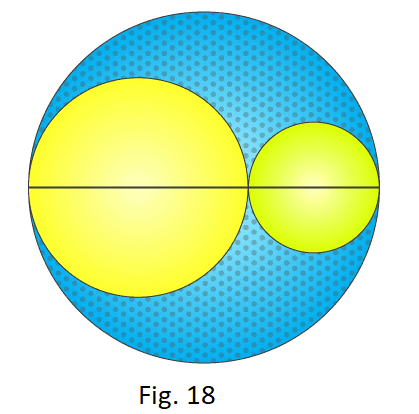Solution:

It is given that, diameter of the big circle = 18 cm

Radius of the big circle = 9 cm

Area of the big circle, A = π r2 = π (9)2 = 81π cm2

Let d1 = (2/3) × 18 = 12 cm

r1 = 6 cm

Area of the circle, A1 = π r2 = π (6)2 = 36π cm2

d2 = (1/3) × 18 = 6 cm

r2 = 3 cm

Area of the circle, A2 = π r2 = π (3)2 = 9π cm2

Area of the shaded portion = A – (A1 + A2)

Area of the shaded portion = 81π – (36π + 9π) = 36π cm2

17. In Fig. 19, the radius of quarter circular plot taken is 2 m and radius of the flower bed is 2 m. Find the area of the remaining field.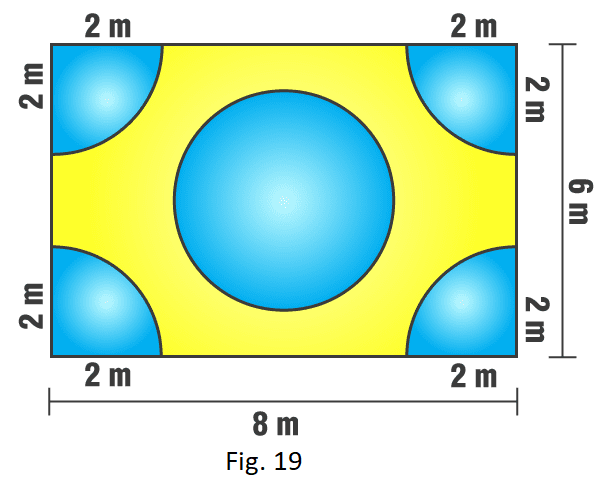Solution:

Given that Radius of flower bed = 2 m

Area of flower bed = π r2 = π (2)2 = 4π

Radius of the quarter circular plot = 2 m

Area of the quarter circular plot = (π r2)/4

Area of 4 quarter circular plots = 4 × (π r2)/4

= π r

= π (2)2

= 4π

We know that area of the rectangular region = Length x Breadth

Area of the rectangular region = 8 x 6 = 48 m2

Area of the remaining field = Area of the rectangular region – (Area of 4 quarter circular plots + Area of the flower bed)

Area of the remaining field = 48 – (4π + 4π)

= 48 – 25.12

= 22.88 m2

18. Four equal circles, each of radius 5 cm, touch each other as shown in Fig. 20. Find the area included between them. (Take π = 3.14).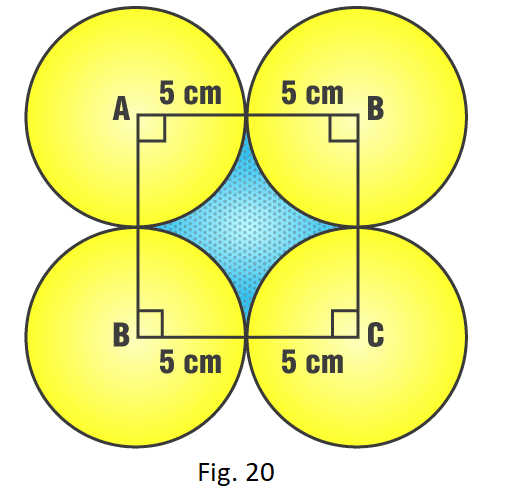Solution:

From the figure we can see that,

Side of the square = 10 cm

We know that area of the square = side x side = 10 x 10 = 100 cm2

Radius of the quarter circle = 5 cm

Area of the quarter circle = (π r2)/4

Area of 4 quarter circle = 4 × (π r2)/4

= π r

= 3.14 × (5)2

= 78.5 cm2

Area included in the quarter circle = Area of the square – Area of the four quarter circles

Area included in them = (100 – 78.5) cm

= 21.5 cm2

19. The area of circle is 100 times the area of another circle. What is the ratio of their circumferences?

Solution:

Let the area of the circles be A1 and A2 and their circumference be c1 and crespectively.

According to the question it is clear that A1 = 100 A2

⇒ π (r1)2 = 100 × π (r2)2

⇒ r1 = 10 r2

⇒ r1/r2 = 10/1 … (i)

Finding the ratios of the circumference;

C1: C2 = 2πr1:  2πr2

C1/C2 = (2πr1)/ (2πr2)

C1/C2 = r1/r2

Putting the value of r1/rfrom equation (i)

C1/C2 = 10/1

C1: C2 = 10: 1

Hence, the ratio of their circumferences is 10: 1.